## Model setting

Since equation () is independent on actual pressure field values, but only on its gradients, we can formally simplify the boundary condition at the outlet by adding a certain constant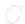to the right hand side of the prescription () in order to get mean value of the outlet pressure equal to zero and not to hydrostatic presure at the center of mass of the outlet surface. If we do this, we have to amend the inlet boundary condition () the same way, of course. We can find easily that the constanthas following value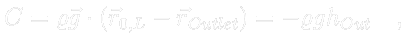(3.29)
where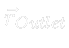is a position vector of the centre of mass of the outlet surface. Hence prescription () changes to(3.30)
and prescription () to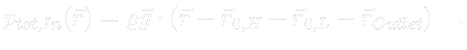(3.31)
A mean value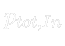of the total pressure at the inlet surface now gives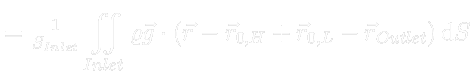(3.32)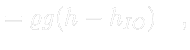(3.35)
where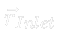is a position vector of the center of mass of the inlet surface and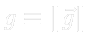. We can see that in this model setting there is no need to know the position of a turbine to the water level. It suffices to know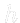and take the measurement of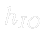.